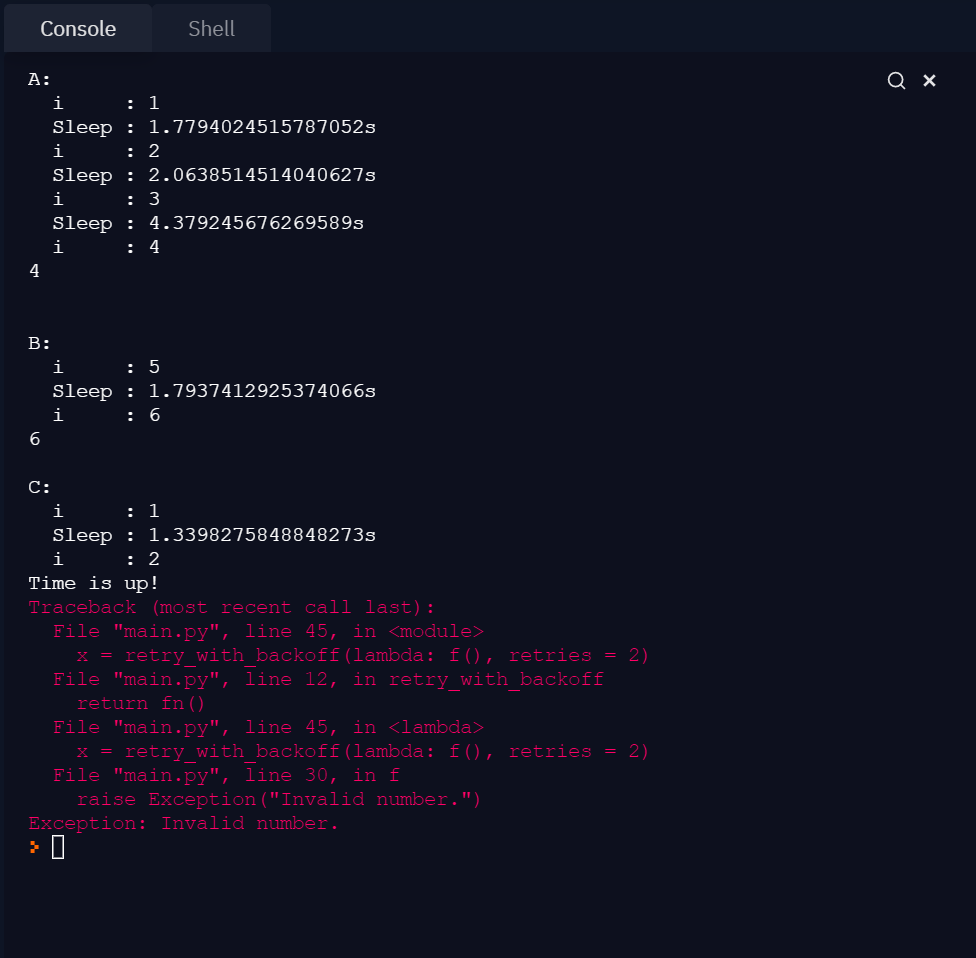# Python utility function: retry with exponential backoff

To make a setup more resilient we should allow for certain actions to be retried before they fail. We should not "hammer" our underlying systems, so it is wise to wait a bit before a retry (exponential backoff). Let's see how we can make a function that implements a "retry and exponential backoff". Note: this only works if actions are idempotent and you can afford to wait.

## Backoff & retry

Let's create a function that retries when an exception is raised. I've added typings, if you need something without typings, look here.

``````import random, time
from typing import TypeVar, Callable

T = TypeVar('T')

def retry_with_backoff(
fn: Callable[[], T],
retries = 5,
backoff_in_seconds = 1) -> T:
x = 0
while True:
try:
return fn()
except:
if x == retries:
raise

sleep = (backoff_in_seconds * 2 ** x +
random.uniform(0, 1))
time.sleep(sleep)
x += 1
``````

De default number of retries is 5.

### Exponential backoff

So what is exponential backoff? Wikipedia says:

In a variety of computer networks, binary exponential backoff or truncated binary exponential backoff refers to an algorithm used to space out repeated retransmissions of the same block of data, often to avoid network congestion.

Source: Wikipedia

I've ended up implementing the algorithm specified by Google Cloud IOT Docs: Implementing exponential backoff. The default backoff time is 1 second. So when the function call fails, it will retry 5 times: after +1, +2, +4, +8 and +16 seconds. If the call still fails, the error will be raised.

## Visual example

To test our resilient setup, we need a function that sometimes throws an exception:

``````import random, time
from typing import TypeVar, Callable
T = TypeVar('T')

def retry_with_backoff(
fn: Callable[[], T],
retries = 5,
backoff_in_seconds = 1) -> T:
x = 0
while True:
try:
return fn()
except:
if x == retries:
print("Time is up!")
raise

sleep = (backoff_in_seconds * 2 ** x +
random.uniform(0, 1))
print("  Sleep :", str(sleep) + "s")
time.sleep(sleep)
x += 1

i=0

def f() -> int:
global i
i = i + 1
print("  i     :", i);
if i < 4 or i % 2 != 0:
raise Exception("Invalid number.")
return i

# should  sleep 3 times
print("A:")
x = retry_with_backoff(f)
print(x, "\n\n")

# should sleep 1 time
print("B:")
x = retry_with_backoff(lambda: f())
print(x, "\n")

# should crash after 2 retries
print("C:")
i = 0
x = retry_with_backoff(lambda: f(), retries = 2)``````

When we execute the code, we see the retry and backoff (sleep) in action:Example A fails 3 times, Example B fails 1 time and example C will not recover within 2 times.

## A decorator?

You can also implement this mechanism as a decorator. The code for the decorator looks like this:

``````def retry_with_backoff(retries = 5, backoff_in_seconds = 1):
def rwb(f):
def wrapper(*args, **kwargs):
x = 0
while True:
try:
return f(*args, **kwargs)
except:
if x == retries:
raise

sleep = (backoff_in_seconds * 2 ** x +
random.uniform(0, 1))
time.sleep(sleep)
x += 1

return wrapper
return rwb``````

You can implement the decorator like this:

``````@retry_with_backoff(retries=6)
def f() -> int:
global i
i = i + 1
print("  i     :", i);
if i < 6 or i % 2 != 0:
raise Exception("Invalid number.")
return i``````

I'm not 100% sure if the decorator is the best solution. The main advantage is that you tie the mechanism to your function, so your caller does not need to implement it. But that is also its weakness, your caller cannot influence the defaults you've set. It heavily depends on your use case if you want to use a decorator.

## Conclusion

You see: it is not so hard to implement retry and exponential backoff in Python. It will make your setup way more resilient!

## Without typings

If you're not a fan of typings or need something small and simple, you can use this code:

``````import random, time

def retry_with_backoff(fn, retries = 5, backoff_in_seconds = 1):
x = 0
while True:
try:
return fn()
except:
if x == retries:
raise

sleep = (backoff_in_seconds * 2 ** x +
random.uniform(0, 1))
time.sleep(sleep)
x += 1``````
expand_less